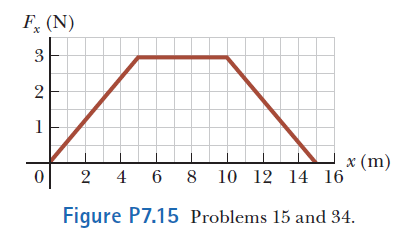# Problem: A particle is subject to a force Fx that varies with position as shown in Figure P7.15. Find the work done by the force on the particle as it moves (a) from x = 0 to x = 5.00 m, (b) from x = 5.00 m to x = 10.0 m, and (c) from x = 10.0 m to x = 15.0 m. (d) What is the total work done by the force over the distance x = 0 to x = 15.0 m?

⚠️Our tutors found the solution shown to be helpful for the problem you're searching for. We don't have the exact solution yet.

###### Problem Details

A particle is subject to a force Fx that varies with position as shown in Figure P7.15. Find the work done by the force on the particle as it moves (a) from x = 0 to x = 5.00 m, (b) from x = 5.00 m to x = 10.0 m, and (c) from x = 10.0 m to x = 15.0 m. (d) What is the total work done by the force over the distance x = 0 to x = 15.0 m?